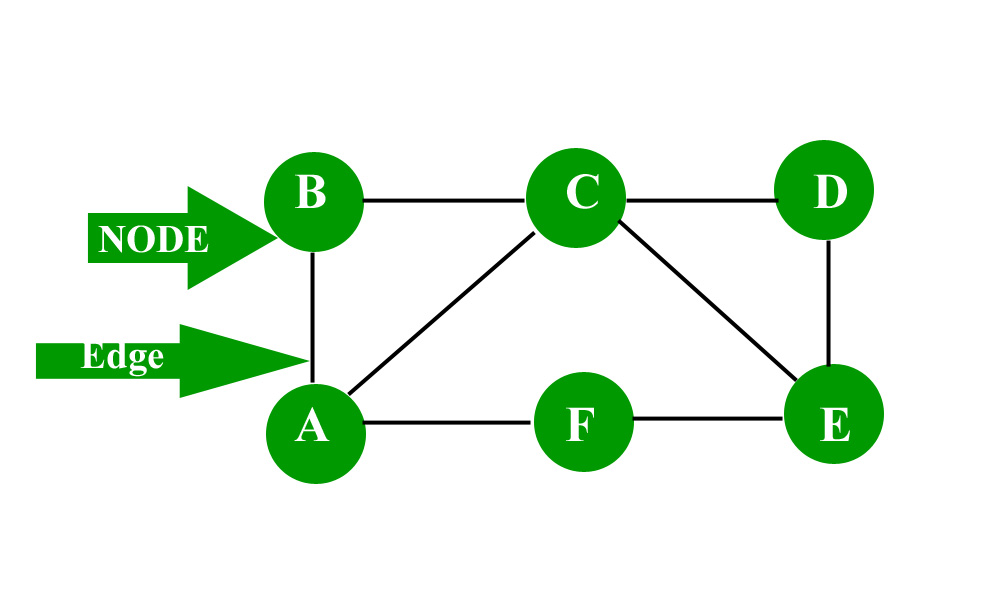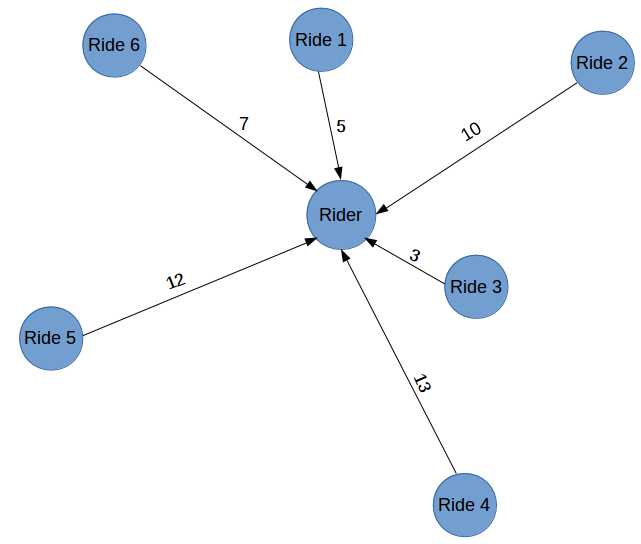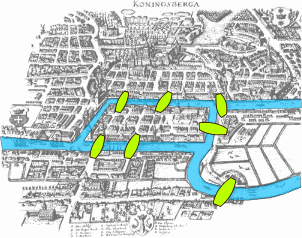# Lecture on Graph Theory:

## Introduction and Applications of Graph Theory

#### What is Graph?

In mathematics, graph theory exists to study graphs, but what exactly is a graph?

Several deffinitions exist:

Graphs are mathematical structures used to study pairwise relationships between objects and entities.

A graph is a structure with a set of objects, these objects can be related to other objects in pairs. The objects correspond to mathematical concepts called vertices, and the connected or related pairs of vertices are called edges.

A graph can also be thought of as: A binary relation (=edges) between elements of a set (=vertices).

Vertices are also often called nodes or points.

Edges can be called links or lines.

Graphs use the terms vertex and edge commonly, while networks usually use node and link.

Examples:

Nodes = vertices (ex: vertices = {A, B, C, D, E})

Edges = links (ex: edges = {AB}, {AC}, {B,C}, {C,E})# Why Graphs?

Suppose you booked an Uber cab. One of the most important things that is critical to Uber’s functioning is its ability to match drivers with riders in an efficient way. Consider there are 6 possible rides that you can be matched with. So, how does Uber allocate a ride to you? We can make use of graphs to visualize how the process of allotting a ride might be.

Which Ride (driver) for the rider makes the most sense?# History of graphs and graph theory

### Leonhard Euler and the Seven Bridges of Kongsberg Problem

History:

Can you take a walk through the town, visting each part of the town and crossing each bridge only once?

Rules: 1. No doubling back. 2. You can only cross a bridge one time. 3. You end at the same place you started.

Simple path vs Euler path

A path in a graph represents a way to get from an origin to a destination node by traversing the edges of the graph.

Simple path: a route around a graph that visits every vertex one is called a simple path.

Euler path: a route around a graph that visits every edge once is called an Euler path.

You can tell which graphs have a Euler path by counting how many vertices have an odd degree. The number of vertices of odd degree must be either zero or two, if not it is not a Euler path.

Two vertices are adjacent if they share a common edge (an edge joins the vertices).

The degree of a vertex is the total number of vertices that are adjacent to the vertex.

Before computers graph theory could become quite complex depending on the application.

Recently the use of graphs and graph theory have become increasingly popular due to advancements in computing power and applications in diverse fields.# Why study graph theory?

1. Graphs provide a better way of dealing with abstract concepts like relationships and interactions. They also offer an intuitively visual way of thinking about these concepts. Graphs also form a natural basis for analyzing relationships in a Social context

2. Graph Databases have become common computational tools and alternatives to SQL (structured query language) and NoSQL databases

3. Graphs are used to model analytics workflows in the form of DAGs (Directed acyclic graphs)

4. Some Neural Network Frameworks also use DAGs to model the various operations in different layers

5. Graph Theory concepts are used to study and model Social Networks, Fraud patterns, Power consumption patterns, Virality and Influence in Social Media. Social Network Analysis (SNA) is probably the best known application of Graph Theory for Data Science

6. It is used in Clustering algorithms – Specifically K-Means

7. System Dynamics also uses some Graph Theory concepts – Specifically loops

8. Path Optimization is a subset of the Optimization problem that also uses Graph concepts

9. From a Computer Science perspective – Graphs offer computational efficiency. The Big O complexity for some algorithms is better for data arranged in the form of Graphs (compared to tabular data)

# Key terms and concepts

Edges = lines or links, edges can have directions, signs, weights, functional equations, or locations.

Vertices = nodes or points, can have labels, weights, or locations.

Directed Graph = a graph that is made up of a set of vertices connected by edges, where the edges have a direction associated with them

Undirected Graph = a graph with a set of vertices or nodes that are connected, where all the edges are bidirectional, sometimes called an undirected network

Average path length = average number of steps along the shortest paths for all possible pairs of network nodes, measure of efficiency of information or mass transport on a network

Breadth first search (BFS) = algorithm that traverses a graph data structure, starts on a node and explores all neighbor nodes before moving to nodes at the next depth level

Depth first search (DFS) = similar to BFS, starts at a node on a graph data structure, searches as far as possible along each branch before backtracking